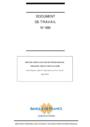# Working Paper Series no. 490: Specification Analysis of International Treasury Yield Curve Factors

#### Abstract

We show how to compute patterns of variation over time, both among and within countries, that determine the international term structure of interest rates, using maximum likelihood within a linear Gaussian state-space framework. The simultaneous estimation of common factors (shared by all countries) and local factors (specific to one country) requires development of a normalization procedure beyond that of ordinary factor analysis. By jointly estimating common and local factors we avoid sequential estimation effects that may explain the lack of agreement in the multi-country term structure literature regarding not only the total number of latent factors required to explain the joint dynamics of yield curves, but also the number of common and of local factors. Using data on international yield curves of U.S., Germany, U.K. and Japan from January 1986 to December 2009, we generally find (analyzing yields in level and in difference) that a model with two common factors and three correlated local factors is preferred to a model (of similar complexity) that includes one common factor only or a model with only correlated local factors. In addition, each common factor closely mimics (or is similar to) a local factor extracted from a pure local factor model. We also reach the conclusion that dependence across international yield curves are driven, first, by the instantaneous correlation between local factors of different countries and, then, by the (full) autoregressive matrix of latent factors and by the matrix of common loadings.

Fulvio Pegoraro, Andrew F. Siegel and Luca Tiozzo ‘Pezzoli’
June 2014

Classification JEL : G12, E43, C52.

Keywords : international treasury yield curves, common and local factors, state-space models, EM algorithm, Kalman Filter and Kalman Smoother